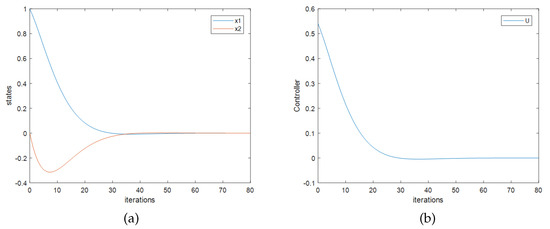Next Article in Journal / Special Issue
A Molecular Force Field-Based Optimal Deployment Algorithm for UAV Swarm Coverage Maximization in Mobile Wireless Sensor Network
Open AccessArticle

# Improved Q-Learning Method for Linear Discrete-Time Systems

byJian Chen 1,†,Jinhua Wang 1,2,† andJie Huang 1,*
1
College of Electrical Engineering and Automation, Fuzhou University, Fuzhou 350108, China
2
Fujian Key Laboratory of New Energy Generation and Power Conversion, Fuzhou 350108, China
*
Author to whom correspondence should be addressed.
These authors contributed equally to this work.
Processes 2020, 8(3), 368; https://doi.org/10.3390/pr8030368
Received: 5 February 2020 / Revised: 16 March 2020 / Accepted: 16 March 2020 / Published: 22 March 2020
In this paper, the Q-learning method for quadratic optimal control problem of discrete-time linear systems is reconsidered. The theoretical results prove that the quadratic optimal controller cannot be solved directly due to the linear correlation of the data sets. The following corollaries have been made: (1) The correlation of data is the key factor in the success for the calculation of quadratic optimal control laws by Q-learning method; (2) The control laws for linear systems cannot be derived directly by the existing Q-learning method; (3) For nonlinear systems, there are some doubts about the data independence of current method. Therefore, it is necessary to discuss the probability of the controllers established by the existing Q-learning method. To solve this problem, based on the ridge regression, an improved model-free Q-learning quadratic optimal control method for discrete-time linear systems is proposed in this paper. Therefore, the computation process can be implemented correctly, and the effective controller can be solved. The simulation results show that the proposed method can not only overcome the problem caused by the data correlation, but also derive proper control laws for discrete-time linear systems. View Full-Text
Show FiguresFigure 1

MDPI and ACS Style

Chen, J.; Wang, J.; Huang, J. Improved Q-Learning Method for Linear Discrete-Time Systems. Processes 2020, 8, 368.